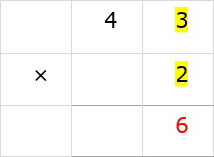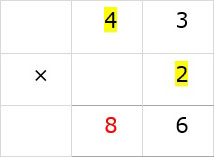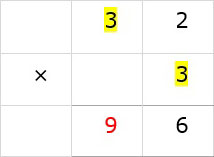# Multiplication Without Carry

#### Complete Python Prime Pack

9 Courses     2 eBooks

#### Artificial Intelligence & Machine Learning Prime Pack

6 Courses     1 eBooks

#### Java Prime Pack

9 Courses     2 eBooks

Multiply 43 x 2

### Solution

Step 1:

First we multiply the ones digit in 43 with 2

i.e., 3 x 2 = 6. We write down 6 below ones column.Step 2:

Next we multiply the tens digit in 43 with 2

i.e., 4 x 2 = 8. We write down 8 below tens column.Step 3:

So 43 × 2 = 86

Multiply 32 x 3

### Solution

Step 1:

First we multiply the ones digit in 32 with 3

i.e., 2 x 3 = 6. We write down 6 below ones column.Step 2:

Next we multiply the tens digit in 32 with 3

i.e., 3 x 3 = 9. We write down 9 below tens column.Step 3:

So 32 × 3 = 96